## Tamilnadu Samacheer Kalvi 6th Maths Solutions Term 1 Chapter 4 Geometry Ex 4.1

Question 1.
Fill in the blanks.
(i) A line through two endpoints ‘A’ and ‘B’ is denoted by ______
(ii) Aline segment from point ‘B’ to point ‘A’ is denoted by ______
(iii) A ray has ______ endpoint(s).
Solution:
(i) $$\overleftrightarrow { AB }$$
(ii) $$\bar { BA }$$
(iii) one

Question 2.
How many line segments are there in the given line? Name them.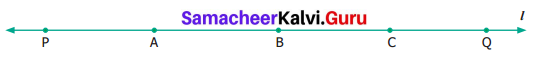Solution:
(i) There are 10 line segments.
(ii) They are $$\overline{\mathrm{PA}}, \overline{\mathrm{AB}}, \overline{\mathrm{BC}}, \overline{\mathrm{CQ}}, \overline{\mathrm{PB}}, \overline{\mathrm{AC}}, \overline{\mathrm{BQ}}, \overline{\mathrm{PC}}, \overline{\mathrm{AQ}} \text { and } \overline{\mathrm{PQ}}$$

Question 3.
Measure the following line segments.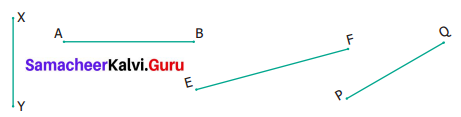Solution:
$$\overline{\mathrm{XY}}=2.4 \mathrm{cm} ; \overline{\mathrm{AB}}=3.4 \mathrm{cm} ; \overline{\mathrm{EF}}=4 \mathrm{cm} ; \overline{\mathrm{PQ}}=3 \mathrm{cm}$$Question 4.
Construct a line segment using ruler and compass.
(i) $$\overline{\mathrm{AB}}=7.5 \mathrm{cm}$$
(ii) $$\overline{\mathrm{CD}}=3.6 \mathrm{cm}$$
(iii) $$\overline{\mathrm{QR}}=10 \mathrm{cm}$$
Solution:
(i) $$\overline{\mathrm{AB}}=7.5 \mathrm{cm}$$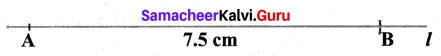Construction:
Step 1: Drawn a line l and marked point A.
Step 2: Measured 7.5 cm using compass placing the pointer at ‘0’ and pencil pointer at 7.5 cm.
Step 3: Placing a compass pointer at A, drawn an arc on l with the pencil pointer. It cut l at B.
Step 4: AB is the required segment of length 7.5 cm.

(ii) $$\overline{\mathrm{CD}}=3.6 \mathrm{cm}$$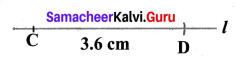Construction:
Step 1: Drawn a line l and marked the point ‘C’ on it.
Step 2: Measured 3.6 cm using a compass by placing the pointer at ‘O’ and pencil at 3.6 cm
Step 3: Placing pointer at C drawn the arc on ‘l’ with pencil pointer
Step 4: CD is the required line segment of length 3.6 cm

(iii) $$\overline{\mathrm{QR}}=10 \mathrm{cm}$$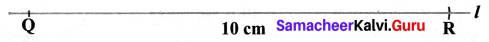Construction:
Step 1: Drawn a line ‘l’ and marked the point Q on it
Step 2: Measured 10 cm using a compass by placing the pointer at ‘0’ and pencil pointer at 10 cm.
Step 3: Placing pointer at Q drawn the arc on ‘l’ with pencil pointer and named the point R
Step 4: QR is the required line segments of 10 cm.

Question 5.
From the given figure
(i) identify the parallel lines
(ii) identify the intersecting lines
(iii) name the points of intersection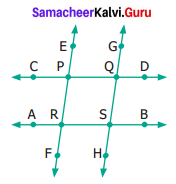Solution:
(i) Parallel lines:
(a) $$\overrightarrow{\mathrm{CD}} \text { and } \overrightarrow{\mathrm{AB}}$$
(b) $$\overrightarrow{\mathrm{EF}} \text { and } \overrightarrow{\mathrm{GH}}$$
(ii) Intersecting lines:
(a) $$\overrightarrow{\mathrm{CD}} \text { and } \overrightarrow{\mathrm{EF}}$$
(b) $$\overrightarrow{\mathrm{CD}} \text { and } \overrightarrow{\mathrm{GH}}$$
(c) $$\overrightarrow{\mathrm{AB}} \text { and } \overrightarrow{\mathrm{EF}}$$
(d) $$\overrightarrow{\mathrm{AB}} \text { and } \overrightarrow{\mathrm{GH}}$$
(iii) Point of Intersection:
P, Q, R and S are the points of Intersection.Question 6.
From the given figure, name the
(i) parallel lines
(ii) intersecting lines
(iii) points of Intersection.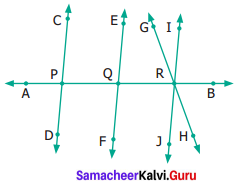Solution:
(i) Parallel lines:
$$\overrightarrow{\mathrm{CD}} \text { and } \overrightarrow{\mathrm{EF}}$$; $$\overrightarrow{\mathrm{CD}} \text { and } \overrightarrow{\mathrm{IJ}}$$; $$\overrightarrow{\mathrm{EF}} \text { and } \overrightarrow{\mathrm{IJ}}$$ are parallel lines.
(ii) Intersecting lines:
(a) $$\overrightarrow{\mathrm{AB}} \text { and } \overrightarrow{\mathrm{CD}}$$
(b) $$\overrightarrow{\mathrm{AB}} \text { and } \overrightarrow{\mathrm{EF}}$$
(c) $$\overrightarrow{\mathrm{AB}} \text { and } \overrightarrow{\mathrm{GH}}$$
(d) $$\overrightarrow{\mathrm{AB}} \text { and } \overrightarrow{\mathrm{IJ}}$$
(e) $$\overrightarrow{\mathrm{GH}} \text { and } \overrightarrow{\mathrm{IJ}}$$
Points of Intersection:
P, Q and R are the points of intersection.

Question 7.
From the given figure, name
(i) all pairs of parallel lines
(ii) all pairs of intersecting lines
(iii) pair of lines whose point of intersection is ‘ V’
(iv) point of intersection of the lines ‘l2‘ and ‘l3
(v) point of intersection of the lines ‘l1‘ and ‘l5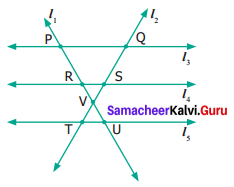Solution:
(i) Pairs of parallel lines:

• l3 and l4
• l4 and l5
• l3 and l5

(ii) Pairs of intersecting lines:

• l1 and l2
• l1 and l3
• l1 and l4
• l1 and l5
• l2 and l3
• l2 and l4
• l2 and l5

(iii) l1 and l2 intersect at ‘V’
(iv) point of intersection of the lines ‘l2‘ and; l5‘ is ‘Q’
(v) point of intersection of the lines ‘l1‘ and ‘l5‘ is ‘U’Objective Type Questions

Question 8.
The number of line segments in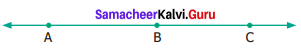is
(a) 1
(b) 2
(c) 3
(d) 4
Solution:
(c) 3
Hint: $$\overline{\mathrm{AB}}, \overline{\mathrm{AC}}, \overline{\mathrm{BC}}$$

Question 9.
A line is denoted as
(a) AB
(b) $$\overrightarrow{AB}$$
(c) $$\overleftrightarrow {AB}$$
(d) $$\overline { AB }$$
Solution:
(c) $$\overleftrightarrow {AB}$$